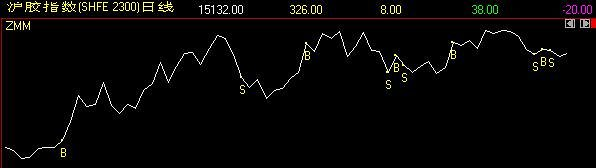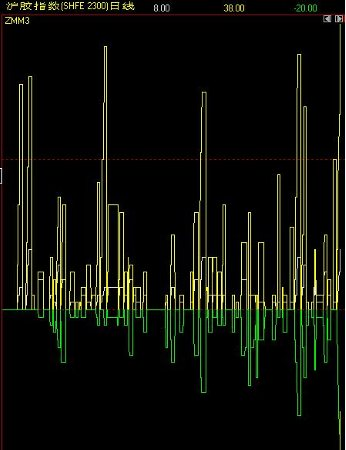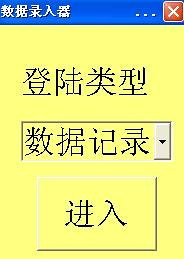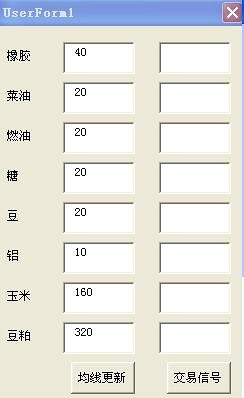# 失败指标雏形

（此文是后来整理的，其中的三个连续失败XX，是后来失败指标的雏形）

系统的基础已经定了（假设上面的改动最终能使得策略与目标匹配）
接下来在基础之上，我要再为主观构建一个层次，或者说是空间。这个层次会有很多限制，包括时间（什么时候开放主观权限），资金量（主观权限的额度），目标匹配（主观操作的目的）等等。
暂时有一个概念，和gu兄提及过，就是“交易别人的资金曲线”。具体呢，就是找一个简单的趋势跟踪系统，独立观察它在各个市场的表现，然后采取相应的主观策略。策略主要可以有两类：1是盈冲，比如统计这个独立系统的“风报比=平均单比亏损幅度/平均单比盈利”，对这个风报比做长短两根X、Y日均线平滑，那么金叉主观入场==实现赢冲。2是输冲（我对这个更喜欢），比如统计这个独立系统的下面这些指标：BB:=BARPOS;
B:=IF(CLOSE>MA(CLOSE,20),1,0);
S:=IF(CLOSE<MA(CLOSE,20),1,0);
SD:=BARSLAST(REF(B,1));
BD:=BARSLAST(REF(S,1));
SR:=IF(S=1&&BB>50&&B=0&&REF(S,1)=0,CLOSE/REF(CLOSE,BD)-1,0);
SR1:=IF(S=1&&BB>50&&REF(S,1)=0,CLOSE-REF(CLOSE,BD),0);
SR2:=IF(BB>50,CLOSE/REF(CLOSE,BD)-1,0);
LASTS:=IF(ISLASTBAR,CLOSE/REF(CLOSE,BD)-1,0);
BR:=IF(B=1&&BB>50&&S=0&&REF(B,1)=0,REF(CLOSE,SD)/CLOSE-1,0);
BR1:=IF(B=1&&BB>50&&REF(B,1)=0,REF(CLOSE,SD)-CLOSE,0);
BR2:=IF(BB>50&&S=0,REF(CLOSE,SD)/CLOSE-1,0);
LASTB:=IF(ISLASTBAR,REF(CLOSE,SD)/CLOSE-1,0);
TR := MAX(MAX((HIGH-LOW),ABS(REF(CLOSE,1)-HIGH)),ABS(REF(CLOSE,1)-LOW));
SSS:=IF(S<>REF(S,1),0,SD+BD);
ZX:=IF(SR>0&&S<>REF(S,1),1,0);
ZC:=IF(BR>0&&S<>REF(S,1),1,0);
ZZ:=ZX+ZC;
ZD:=BARSLAST(ZZ)-SSS;
AA:=IF(ZD>0,0,1);